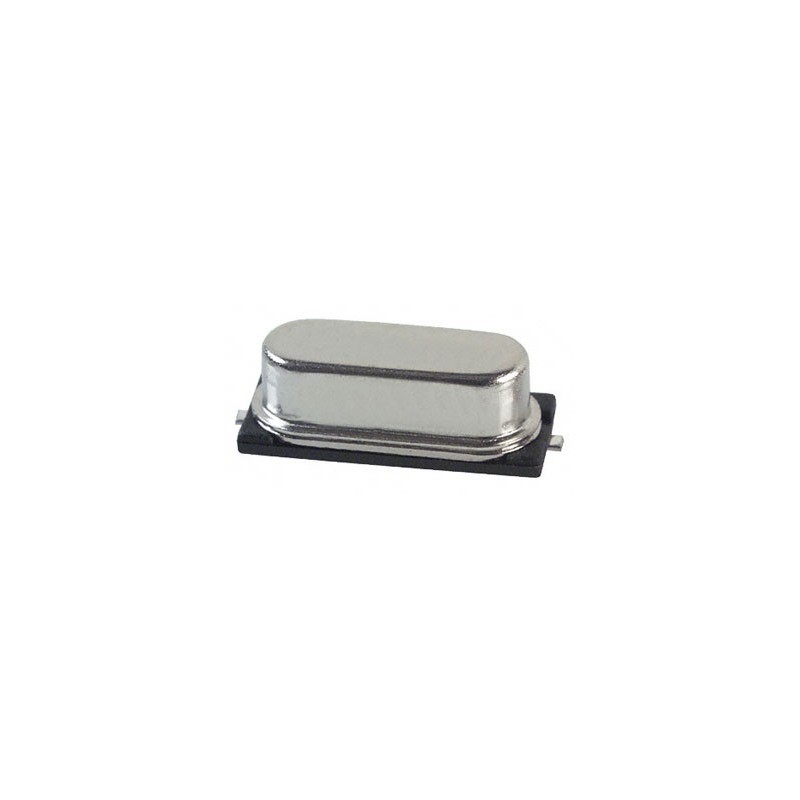﻿Zobacz większe

# Boundary Value Problems

David Powers

ID: 170804

For international customers:

Boundary Value Problems in Kamami.com

### Więcej informacji

Boundary Value Problems is the leading text on boundary value problems and Fourier series for professionals and students in engineering, science, and mathematics who work with partial differential equations.

In this updated edition, author David Powers provides a thorough overview of solving boundary value problems involving partial differential equations by the methods of separation of variables. Additional techniques used include Laplace transform and numerical methods. Professors and students agree that Powers is a master at creating examples and exercises that skillfully illustrate the techniques used to solve science and engineering problems.

• New animations and graphics of solutions, additional exercises and chapter review questions on the web

• Nearly 900 exercises ranging in difficulty from basic drills to advanced problem-solving exercises

• Many exercises based on current engineering applications

• An Instructor's Manual and Student Solutions Manual are available separately

Preface
Chapter 0 -- Differential Equations
Chapter 1 -- Fourier Series and Integrals
Chapter 2-- The Heat Equation
Chapter 3 -- The Wave Equation
Chapter 4 -- The Potential Equation
Chapter 5 -- Higher Dimensions & Other Coordinates
Chapter 6 -- Laplace Transform
Chapter 7 -- Numerical Methods
Bibliography
Appendix